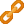#Apply Model (RapidMiner Studio Core)

## Synopsis

This Operator applies a model on an ExampleSet.

## Description

A model is first trained on an ExampleSet by another Operator, which is often a learning algorithm. Afterwards, this model can be applied on another ExampleSet. Usually, the goal is to get a prediction on unseen data or to transform data by applying a preprocessing model.

The ExampleSet upon which the model is applied, has to be compatible with the Attributes of the model. This means, that the ExampleSet has the same number, order, type and role of Attributes as the ExampleSet used to generate the model.

## Differentiation

###Group Models

If you want to apply several models in a row you can use the Group Models Operator. This is helpful if you for example want to apply preprocessing models before applying a prediction model.

## Input

•Model (Model)

This port expects a model. The number, order, type and role of Attributes of the ExampleSet on which this model was trained has to be consistent with the ExampleSet on the unlabeled data input port.

•Unlabelled data (Data Table)

This port expects an ExampleSet. The number, order, type and role of Attributes of this ExampleSet has to be consistent with ExampleSet on which the model delivered to the model input port was trained.

## Output

•labelled data (Data Table)

The ExampleSet delivered from this port is changed by means of the model. For the case of predictions, new Attributes like 'prediction(Label)' and 'confidence(Value)' are added. Applying preprocessing models updates the existing ExampleSet.

•model (Model)

The input model is passed without changing to the output through this port.

## Parameters

• application_parameters

This parameter can change the settings of certain models before they are applied to provided ExampleSet. This is only possible for a few Operators and can be considered a legacy option.

Range:
• create_view

If the model applied at the input port supports Views, it is possible to create a View instead of changing the underlying data. If this option is checked, the application of the model is delayed until the transformations are needed. Most models no longer support Views and it can be considered a legacy option.

Range:

## Tutorial Processes

### Train and apply a linear regression model

In this tutorial Process, a model is created within the training of a linear regression.

This tutorial Process first trains a linear regression model on the 'Polynomial' ExampleSet. Afterwards, the model is applied on an unlabelled 'Polynomial' ExampleSet. The resulting output ExampleSet has a new Attribute: 'prediction(label)'.